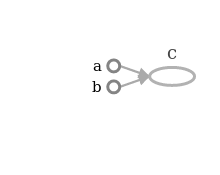# TensorFlow - Exercise 2 - Variables in TensorFlow

Exercise : Generate a random array of size 100 and find mean of z as obtained from below equations: 1. y = xx + 5x 2. z = yy

Implement both the equations seperately and * means element wise multiplication and not matrix multiplication. Below are some restrictions for this exercise:

1. y should be of type tf.Variable()
2. Use tf.random_normal() to get random tensor of size 100. Use seed as 12.

# Caution : Solution for exercise is below. Please try solving problem by yourself before looking below

``````import tensorflow as tf

# get 100 random tensors. 12 is used as seed.
x = tf.random_normal(, seed=12,name="x")
y = tf.Variable(x*x+5*x,name="y")
z = tf.multiply(y,y,name="z")
zmean = tf.reduce_mean(z)

init_op = tf.global_variables_initializer()

with tf.Session() as sess:
sess.run(init_op)
zout = sess.run(zmean)
print(zout)
``````
``````27.7974
``````

Summary of what each line does in above code:

Line 1 : Import tensorflow library with alias tf.

Line 4 : Generate random tensor of size 100.

Line 5 : Create a variable y. Initial value of y is given as xx+5x.

Line 6 : Multiply y and y.

Line 7 : Calculate mean of z.

Line 9 : When you launch the graph, variables have to be explicitly initialized before you can run Ops that use their value. You can initialize a variable by running its initializer.

Line 11 : Create a new session to run our graph.

Line 12 : Run the operation to initialize global variables.

Line 13 : Run graph to calculate value of zmean. This will calculate x, y and finally zmean.

For your own unerstanding you can try looking at graph of this code in tensorboard.

# TensorFlow - Exercise 1 - Sum of two numbers

Exercise : Sum of two numbers

Given two number say 5 and 15 find the sum of these two numbers. You are given the numbers to add in advance and the numbers won’t change.

Excepted output 20

# Caution : Solution for exercise is below. Please try solving problem by yourself before looking below

``````# Code starts here
import tensorflow as tf

# As we are told that numbers won't change
# so it's safe to assume it as tensorflow constant
a = tf.constant(5,name="a")
b = tf.constant(15,name="b")

# Now we have to add a and b.
# tenorflow provides add function for same.

# Tensor flow creates graph and doesn't run the graph till
# you run the graph in a session.
# So if we try printing value of c at this point
# we will get the output as a tensor and not actual value of c
# This is because value of c is not computed till we demand
# value of c, (and this is done by sess.run() )
print("Value of c before running tensor:",c)
``````
``````Value of c before running tensor: Tensor("c_5:0", shape=(), dtype=int32)
``````
``````# A new session is created using tf.Session() call.
sess = tf.Session()

# now we need to run graph we created
# c is passed as input to run as we need
# to run graph till value of c is obtained.
output = sess.run(c)
print("Value of c after running graph:",output)

# Once we are done we need to close the session.
sess.close()

# code ends here
``````
``````Value of c after running graph: 20
``````

While learning tensorflow I kept hearing that tensorflow creates graph, but it was hard to visualize. I used to wonder if I can see the graph which tensorflow creates. And the answer is YES. We can actually see the graph created by tensorflow.

Tensorflow provides a tool called Tensorboard which can be used to see the graph created by tensorflow. Please google and learn about tensorboard in detail. Refer: Tensorboard for details on same.

For now, Let’s check the graph created for above program by tensorboard. The graph created for above program is:As it can be seen in the graph that c is dependent on a and b. So when we execute sess.run(c) tensorflow evalutes c based on a and b. Tensor a and b are evaluted first and then c is computed based on value of a and b.

Let’s try last thing for this exercise. What happens if we define d and don’t use value of d at all. Consider code below

``````import tensorflow as tf

a = tf.constant(5,name="a")
b = tf.constant(15,name="b")
``````20